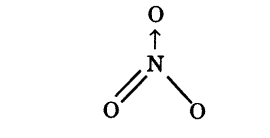# Calculate the oxidation number of sulphur chromium and nitrogen in \${{H}_{2}}\$S\${{O}_{5}}\$, \${{Cr}_{2}}\$\${{O}_{7}}^{2-}\$, N\${{O}_{3}}^{-}\$. Suggest structure of these compounds. Count for the fallacy

Oxidation No. of S in \${{H}{2}}S{{O}{5}} can be calculated byunderstanding its structure. (i) By conventional method {{H}{2}}S{{O}{5}} or 2(+ 1) + x + 5(- 2) = 0 or x = + 8 (wrong) But this is wrong as the maximum oxidation no. of sulphur cannot be more than + 6, since it has only six valence electrons. <img src="/uploads/db3785/original/2X/f/f5cbbe8724a579ce96db5ad0c015535621469c14.png" width="350" height="185"> in which two oxygen atoms are joined by peroxide linkage. The oxidation no. of S can be calculated as 2(+ 1) + x + 3(- 2) + 2(-1) =0 (for H) (for O-O) +2+x-6-2 =0 or x = + 6 Thus, oxidation no. of S in {{H}{2}}S{{O}{5}} = + 6. (ii) Oxidation no. of Cr in {{Cr}{2}}\$\${{O}{7}}^{2-} By conventional method {{Cr}{2}}\$\${{O}{7}}^{2-} or 2x + 7(- 2)=-2 or x=+6 In this case there is no fallacy. (iii) Oxidation no. of N in N{{O}{3}}^{-} Let Oxidation no. of N in N{{O}{3}}^{-} = x x + 3(-2) =-l x = + 5 Oxidation no. of N in N{{O}{3}}^{-} = + 5 Structure of N{{O}{3}}^{-}\$ ion is